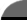Facts By Category:» Physics  » Astronomy  » Chemistry  » Biology  » Mathematics  » Geology  » Engineering  » Medicine  » Science  ScienceIQ Team:»Writers & Editors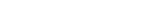xUmp.com
Science Supplies,
 Mathematics: Featured FactsPrime Numbers Mathematics / Number TheoryA prime number is a number that is divisible only by one and by itself. Factors are numbers that can be divided into a number with no remainder. The factors of 18 are the numbers 1, 2, 3, 6, 9, and 18 continue reading this fact Eratosthenes Measured Earth’s Circumference—Centuries Before Columbus Sailed Mathematics / Geometry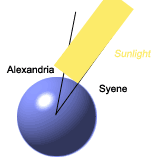Eratosthenes (c. 276 – 194 BC) was born more than 2200 years ago in the Greek city of Cyrene, now a city in the North African country of Libya. (The Greek Empire surrounded much of the Mediterranean S continue reading this fact How To Calculate The Area Of A Circle Mathematics / Geometry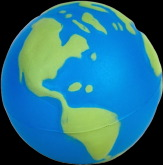A circle is the round counterpart of a square. To find the area of a square, one multiplies the length by the width. A circle doesn't have these, however, so there has to be a different way to calcula continue reading this fact All FactsAlgebra: Geometry: History: Math Function: Number Theory: Topology:
 Home | Privacy Policy | Cookie Policy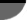Copyright © 2002-2020 ScienceIQ.com - All Rights Reserved